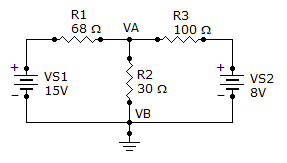# Online Electrical Engineering Test - Electrical Engineering Test 8Loading Test...

Instruction:

• This is a FREE online test. DO NOT pay money to anyone to attend this test.
• Total number of questions : 20.
• Time alloted : 30 minutes.
• Each question carry 1 mark, no negative marks.
• DO NOT refresh the page.
• All the best :-).

1.

When converting 0.16 mA to microamperes, the result is

A.
 16 µAB.
 160 µAC.
 1,600 µAD.
 0.0016 µA2.

The maximum resistance value for a brown, red, yellow, gold resistor is

A.
 126,000B.
 126,600C.
 114,000D.
 132,0003.

The unit of electrical charge is the

A.
 voltB.
 ampereC.
 jouleD.
 coulomb4.

How much voltage is needed to produce 2.5 A of current through a 200resistor?

A.
 50 VB.
 500 VC.
 80 VD.
 8 V5.

The formula for finding resistance when current and voltage are known is R = I/V.

A.
 TrueB.
 FalseExplanation:

Ohm's Law : V = IR

6.

Two resistors are in series: a 5.6 kresistor and a 4.7 kresistor. The voltage drop across the 5.6 kresistor is 10 V. The voltage across the 4.7 kresistor is

A.
 8.39 VB.
 10 VC.
 2.32 VD.
 0 V7.

A series circuit acts as a voltage divider.

A.
 TrueB.
 False8.

Find the node voltage VA.A.
 518 mVB.
 5.18 VC.
 9.56 VD.
 956 mVExplanation:

Reason: The voltage drop through R2 is 5.18v.

9.

When the north poles of two bar magnets are brought close together, there will be

A.
 no forceB.
 a downward forceC.
 a force of attractionD.
 a force of repulsion10.

Ampere-turn is the unit of magnetomotive force (mmf).

A.
 TrueB.
 False11.

A 1 kHz signal has a period of 1 ms.

A.
 TrueB.
 False12.

Five inductors are connected in series. The lowest value is 8 μH. If the value of each inductor is twice that of the preceding one, and if the inductors are connected in order of ascending values, the total inductance is

A.
 8 HB.
 32 μHC.
 64 μHD.
 248 μH13.

If a transformer has 50 turns in the primary winding and 10 turns in the secondary winding, what is the reflective resistance if the secondary load resistance is 250?

A.
 250B.
 25C.
 6,250D.
 62,50014.

In an RC circuit, the impedance is determined by both the resistance and the capacitive reactance combined.

A.
 TrueB.
 False15.

A resistor of 3 k, a 0.05F capacitor, and a 120 mH coil are in series across a 5 kHz, 20 V ac source. What is the impedance, expressed in polar form?

A.
 636B.
 3,769C.
 433D.
 4,33716.

The impedance at the resonant frequency of a series RLC circuit with L = 20 mH, C = 0.02F, and RW = 90is

A.
 0B.
 90C.
 20 kD.
 40 k17.

Referring to Problem 15, if the input voltage is a 6 V sine wave with a dc level of 10 V, what is the output voltage magnitude?

A.
 6 VB.
 597 mVC.
 5.97 VD.
 0 V18.

Referring to Problem 8, assume the input voltage is 15 V dc. What is the output voltage?

A.
 0 VB.
 15 VC.
 7.07 VD.
 10.6 V19.

In an RL low-pass filter, the output voltage is taken across the inductor and the output lags the input.

A.
 TrueB.
 False20.

Norton's theorem gives

A.
 an equivalent current source in parallel with an equivalent impedanceB.
 an equivalent current source in series with an equivalent impedanceC.
 an equivalent voltage source in parallel with an equivalent impedanceD.
 an equivalent voltage source in series with an equivalent impedance